# 1 Getting started

VSN is a method to preprocess microarray intensity data. This can be as simple as

library("vsn")
data("kidney")
xnorm = justvsn(kidney)

where kidney is an ExpressionSet object with unnormalised data and xnorm the resulting ExpressionSet with calibrated and glog$$_2$$-transformed data.

M = exprs(xnorm)[,1] - exprs(xnorm)[,2]

produces the vector of generalised log-ratios between the data in the first and second column.

VSN is a model-based method, and the more explicit way of doing the above is

fit = vsn2(kidney)
ynorm = predict(fit, kidney)

where fit is an object of class vsn that contains the fitted calibration and transformation parameters, and the method predict applies the fit to the data. The two-step protocol is useful when you want to fit the parameters on a subset of the data, e.g. a set of control or spike-in features, and then apply the model to the complete set of data (see Section 7 for details). Furthermore, it allows further inspection of the fit object, e.g. for the purpose of quality assessment.

Besides ExpressionSets, there are also justvsn methods for AffyBatch objects from the affy package and RGList objects from the limma package. They are described in this vignette.

The so-called glog$$_2$$ (short for generalised logarithm) is a function that is like the logarithm (base 2) for large values (large compared to the amplitude of the background noise), but is less steep for smaller values. Differences between the transformed values are the generalised log-ratios. These are shrinkage estimators of the logarithm of the fold change. The usual log-ratio is another example for an estimator1 In statistics, the term estimator is used to denote an algorithm that calculates a value from measured data. This value is intended to correspond to the true value of a parameter of the underlying process that generated the data. Depending on the amount of the available data and the quality of the estimator, the intention may be more or less satisfied. of log fold change. There is also a close relationship between background correction of the intensities and the variance properties of the different estimators. Please see Section 12 for more explanation of these issues.

How does VSN work? There are two components: First, an affine transformation whose aim is to calibrate systematic experimental factors such as labelling efficiency or detector sensitivity. Second, a glog$$_2$$ transformation whose aim is variance stabilisation.

An affine transformation is simply a shifting and scaling of the data, i.e. a mapping of the form $$x\mapsto (x-a)/s$$ with offset $$a$$ and scaling factor $$s$$. By default, a different offset and a different scaling factor are used for each column, but the same for all rows within a column. There are two parameters of the function vsn2 to control this behaviour: With the parameter strata, you can ask vsn2 to choose different offset and scaling factors for different groups (“strata”) of rows. These strata could, for example, correspond to sectors on the array2 See Section 5.2.. With the parameter calib, you can ask vsn2 to choose the same offset and scaling factor throughout3 See Section 10.. This can be useful, for example, if the calibration has already been done by other means, e.g., quantile normalisation.

Note that VSN’s variance stabilisation only addresses the dependence of the variance on the mean intensity. There may be other factors influencing the variance, such as gene-inherent properties or changes of the tightness of transcriptional control in different conditions. These need to be addressed by other methods.

# 2 Running VSN on data from a single two-colour array

The dataset kidney contains example data from a spotted cDNA two-colour microarray on which cDNA from two adjacent tissue samples of the same kidney were hybridised, one labeled in green (Cy3), one in red (Cy5). The two columns of the matrix exprs(kidney) contain the green and red intensities, respectively. A local background estimate4 See Section 12 for more on the relationship between background correction and variance stabilising transformations. was calculated by the image analysis software and subtracted, hence some of the intensities in kidney are close to zero or negative. In Figure 1 you can see the scatterplot of the calibrated and transformed data. For comparison, the scatterplot of the log-transformed raw intensities is also shown. The code below involves some data shuffling to move the data into datasframes for ggplot.

library("ggplot2")
allpositive = (rowSums(exprs(kidney) <= 0) == 0)

df1 = data.frame(log2(exprs(kidney)[allpositive, ]),
type = "raw",
allpositive = TRUE)
df2 = data.frame(exprs(xnorm),
type = "vsn",
allpositive = allpositive)
df = rbind(df1, df2)
names(df)[1:2] = c("x", "y")

ggplot(df, aes(x, y, col = allpositive)) + geom_hex(bins = 40) +
coord_fixed() + facet_grid( ~ type)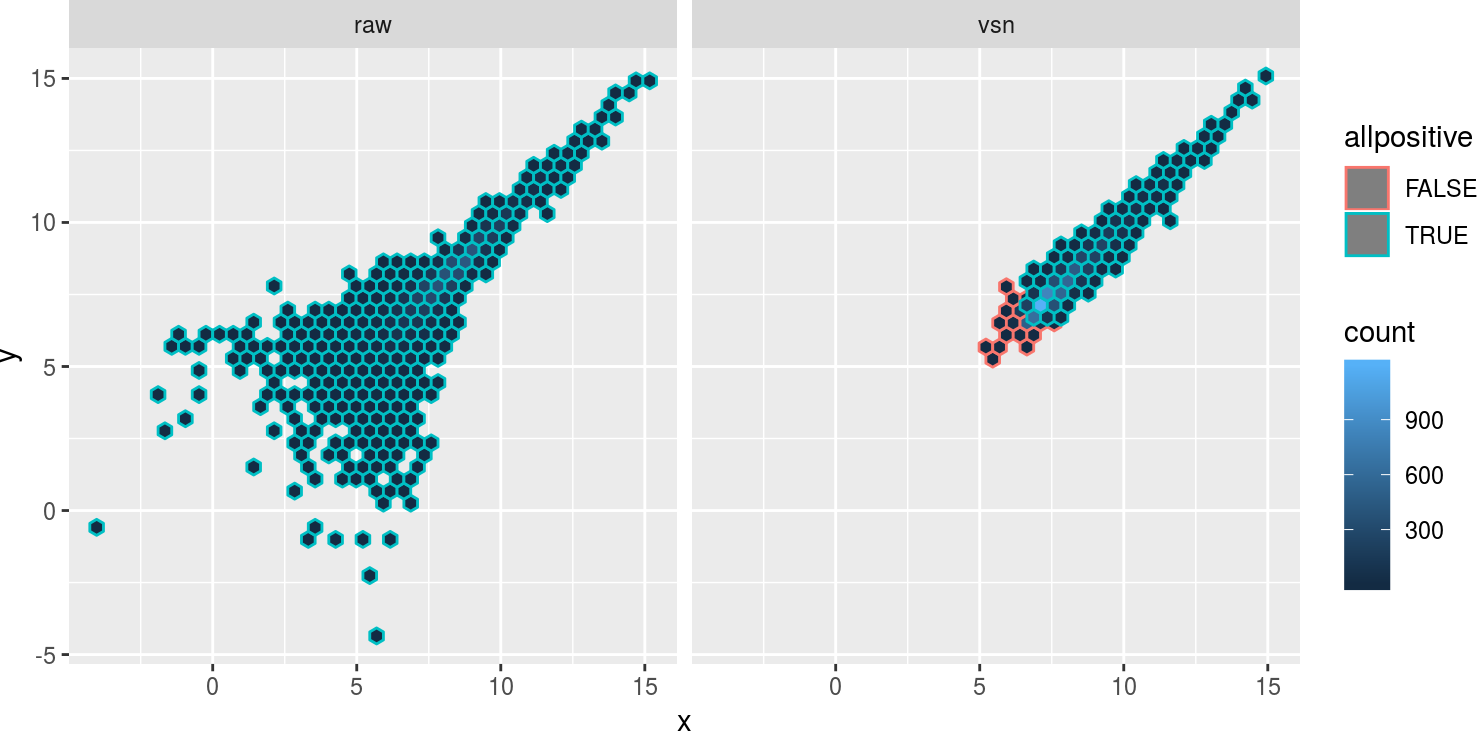Figure 1: Scatterplots of the kidney example data, which were obtained from a two-color cDNA array by quantitating spots and subtracting a local background estimate
a) unnormalised and $$\log_2$$-transformed. b) normalised and transformed with VSN. Panel b shows the data from the complete set of 8704 spots on the array, Panel a only the 7806 spots for which both red and green net intensities were greater than 0. Those spots which are missing in Panel a are coloured in orange in Panel b.

To verify the variance stabilisation, there is the function meanSdPlot. For each feature $$k=1,\ldots,n$$ it shows the empirical standard deviation $$\hat{\sigma}_k$$ on the $$y$$-axis versus the rank of the average $$\hat{\mu}_k$$ on the $$x$$-axis.

$\begin{equation} \hat{\mu}_k =\frac{1}{d} \sum_{i=1}^d h_{ki}\quad\quad \hat{\sigma}_k^2=\frac{1}{d-1}\sum_{i=1}^d (h_{ki}-\hat{\mu}_k)^2 \end{equation}$
meanSdPlot(xnorm, ranks = TRUE)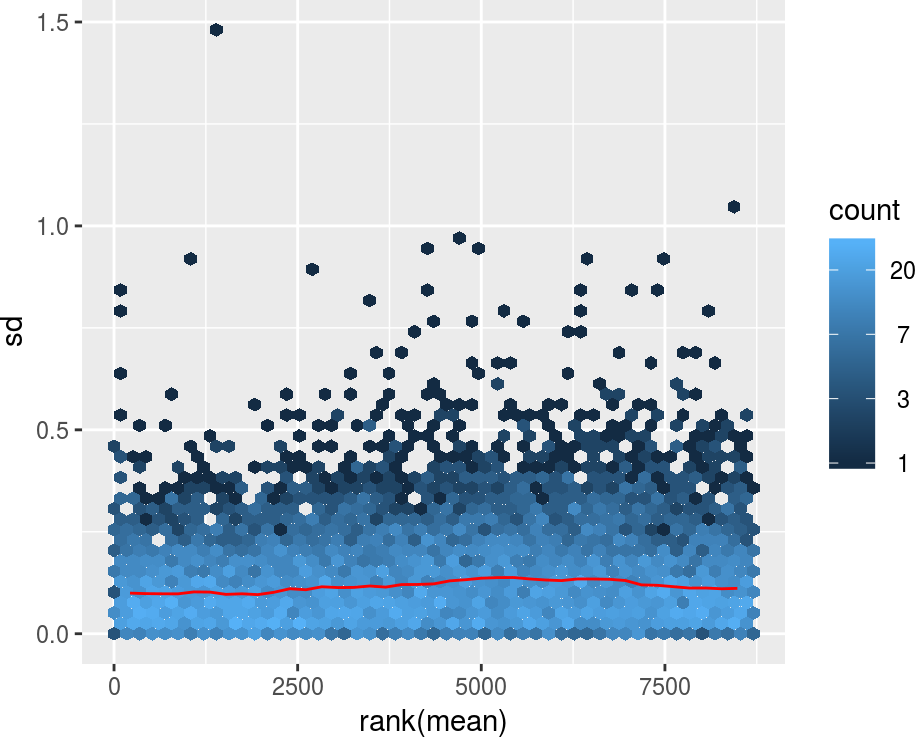Figure 2: Standard deviation versus rank of the mean

meanSdPlot(xnorm, ranks = FALSE)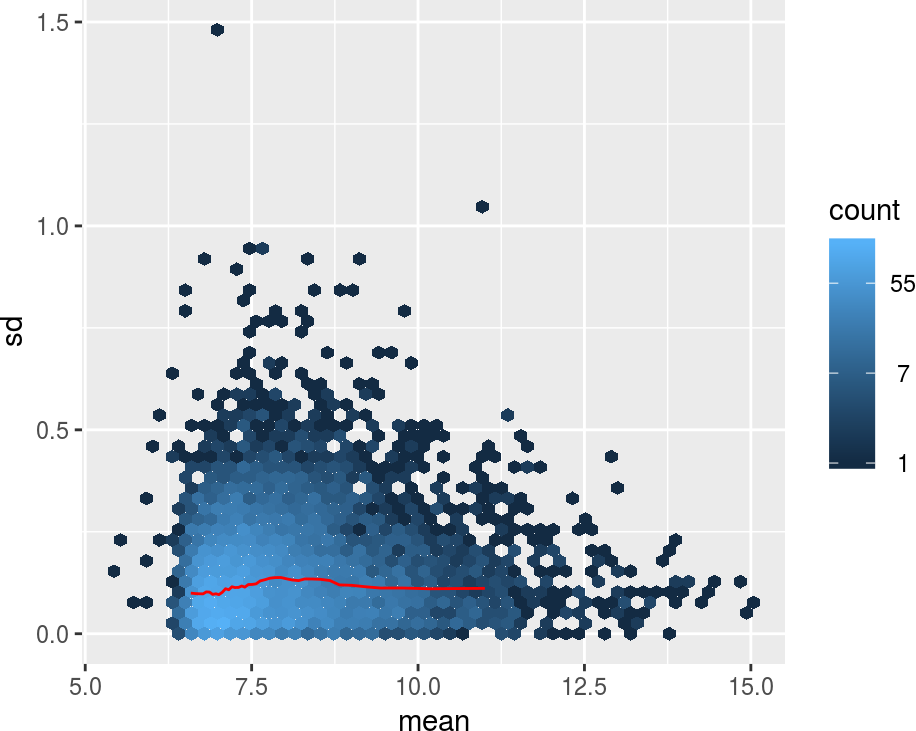Figure 3: Standard deviation versus mean

The red dots, connected by lines, show the running median of the standard deviation5 The parameters used were: window width 10%, window midpoints 5%, 10%, 15%, … . It should be said that the proper way to do is with quantile regression such as provided by the quantreg package—what is done here for these plots is simple, cheap and should usually be good enough due to the abundance of data.. The aim of these plots is to see whether there is a systematic trend in the standard deviation of the data as a function of overall expression. The assumption that underlies the usefulness of these plots is that most genes are not differentially expressed, so that the running median is a reasonable estimator of the standard deviation of feature level data conditional on the mean. After variance stabilisation, this should be approximately a horizontal line. It may have some random fluctuations, but should not show an overall trend. If this is not the case, that usually indicates a data quality problem, or is a consequence of inadequate prior data preprocessing. The rank ordering distributes the data evenly along the $$x$$-axis. A plot in which the $$x$$-axis shows the average intensities themselves is obtained by calling the plot command with the argument ranks=FALSE; but this is less effective in assessing variance and hence is not the default.

The histogram of the generalized log-ratios:

hist(M, breaks = 100, col = "#d95f0e")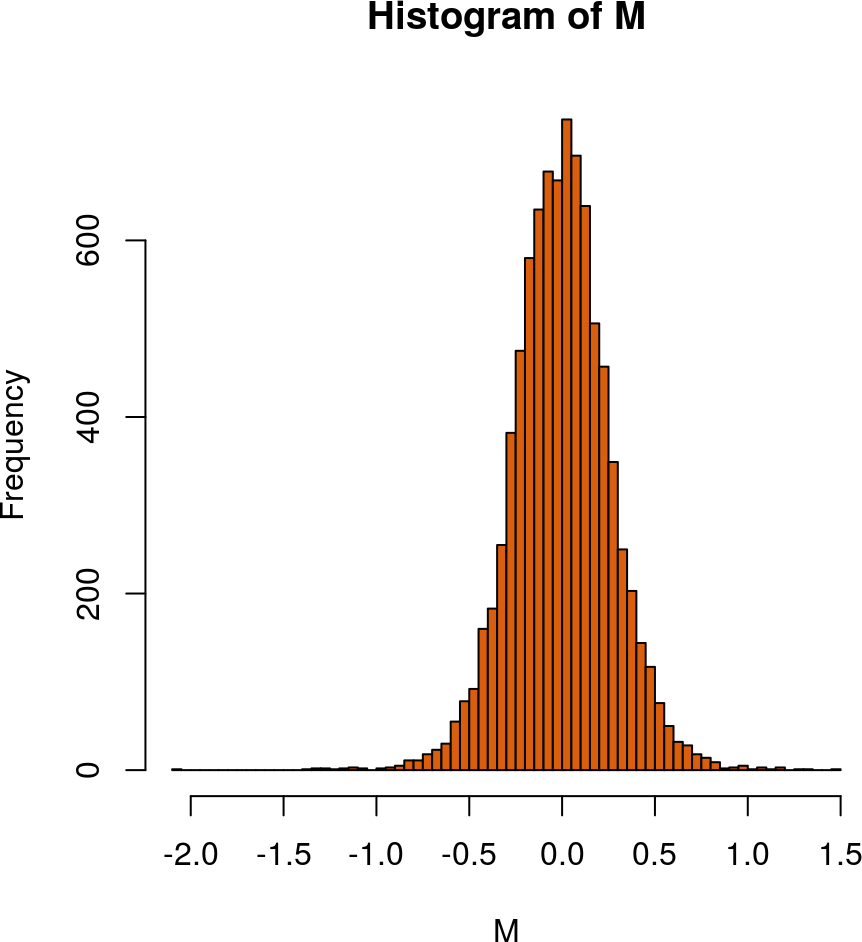Figure 4: Histogram of generalised log-ratios for the kidney example data

# 3 Running VSN on data from multiple arrays (“single colour normalisation”)

The package includes example data from a series of 8 spotted cDNA arrays on which cDNA samples from different lymphoma were hybridised together with a reference cDNA (Alizadeh et al. 2000).

data("lymphoma")
dim(lymphoma)
## Features  Samples
##     9216       16

The 16 columns of the lymphoma object contain the red and green intensities, respectively, from the 8 slides.

pData(lymphoma)
##                      name    sample dye
## lc7b047.reference lc7b047 reference Cy3
## lc7b047.CLL-13    lc7b047    CLL-13 Cy5
## lc7b048.reference lc7b048 reference Cy3
## lc7b048.CLL-13    lc7b048    CLL-13 Cy5
## lc7b069.reference lc7b069 reference Cy3
## lc7b069.CLL-52    lc7b069    CLL-52 Cy5
## lc7b070.reference lc7b070 reference Cy3
## lc7b070.CLL-39    lc7b070    CLL-39 Cy5
## lc7b019.reference lc7b019 reference Cy3
## lc7b019.DLCL-0032 lc7b019 DLCL-0032 Cy5
## lc7b056.reference lc7b056 reference Cy3
## lc7b056.DLCL-0024 lc7b056 DLCL-0024 Cy5
## lc7b057.reference lc7b057 reference Cy3
## lc7b057.DLCL-0029 lc7b057 DLCL-0029 Cy5
## lc7b058.reference lc7b058 reference Cy3
## lc7b058.DLCL-0023 lc7b058 DLCL-0023 Cy5

We can call justvsn on all of them at once:

lym = justvsn(lymphoma)
meanSdPlot(lym)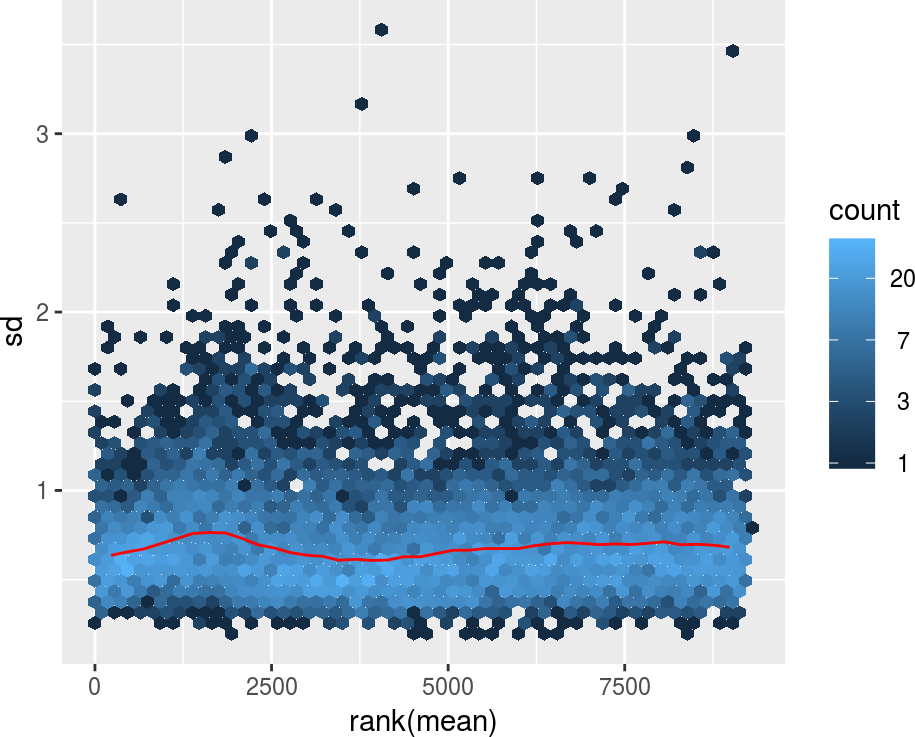Figure 5: Standard deviation versus rank of the mean for the lymphoma example data

We see that the variance stabilisation worked. As above, we can obtain the generalised log-ratios for each slide by subtracting the common reference intensities from those for the 8 samples:

iref = seq(1, 15, by=2)
ismp = seq(2, 16, by=2)
M = exprs(lym)[,ismp]-exprs(lym)[,iref]
A =(exprs(lym)[,ismp]+exprs(lym)[,iref])/2
colnames(M) = lymphoma\$sample[ismp]
colnames(A) = colnames(M)

j = "DLCL-0032"
smoothScatter(A[,j], M[,j], main=j, xlab="A", ylab="M", pch=".")
abline(h=0, col="red")